5 Activities That Will Help Kids to Learn about Measurement

# Measurement Activities for Kids

We measure ingredients while cooking and baking; we measure the size of a T-Shirt we are thinking of purchasing and so many more things. It is one of those math skills that are very much needed in our day to day lives. Teaching kids how to measure is important as it makes them able to go about life with ease.

## What Basic Units of Measurements Should a Kid Know?

Here are some basic units of measurements that children should know:

### 1. Length/Distance

• Inches are used for measuring small lengths such as the length of a paper. An inch is 2.54cm.
• Feet can be used to measure medium-sized objects like the width of a room or a person’s height. 1 foot is 0.3048 meters.
• Yards are used to measure distances of a medium size such as the length of a hockey field. 1 yard equals 0.9144 meters.
• Miles can be used while measuring longer distances like from school to your home. 1 mile is 1.6 kilometres
• Meters are the basic unit used to measure length in the metric system. For smaller measurements, millimetres are used. 1 meter is 1000 millimetres.
• Kilometres are the unit used to measure longer distances. 1 kilometre is 1000 meters.

### 2. Mass/Weight

• Ounces are used to measure smaller mass quantities. 1 ounce is equal to 28.3459 grams.
• Pounds are used to measure the mass of either medium or large objects. It is used to measure the weight of a person. I pound is 16 ounces.
• Tons are used to measure the weight of very large objects. 1 ton is 2000 pounds and 1000 kilograms
• Grams are the basic unit of measurement for weight and mass in the metric system. Smaller objects are measured in milligrams and larger ones in kilograms.

### 3. Volume

• Fluid ounces are used to measure volume in the imperial system.
• Cups are a unit used for medium sized measurements of volume, most commonly used in recipes for cooking. 1 cup is 8 fluid ounces. 1 US cup equals 0.946 metric cups.
• Pints are another unit to measure medium-sized volume. 1 pint is equal to 2 cups
• Quarts are used to measure items that are slightly larger. 1 quart is 4 cups.
• Gallons are used for the measurement of larger volumes like large quantities of water. 1 gallon is 4 quarts and 16 cups.
• A litre is the basic unit of measurement for volume in the metric system. For smaller volumes, a millilitre is used. 1 gallon equals 3.78541 litres, and 1 litre equals 1000 millilitres.

### 4. Temperature

• Degrees are used to measure temperature. It is either measured in Fahrenheit or Celsius.

### 5. Time

• Seconds are the smallest measure of time. There are 60 seconds in one minute
• Minutes are a measure of time. There are 60 minutes in one hour.
• Hours are a medium measurement used to tell the time. There are 24 hours in one day.
• Days, weeks, months and years are used for longer measurements of time.

## Fun Measurement Activities for Children

Here are some fun measurement activities that you can do with your kids:

### 1. Which Jar has More?

If you ever wonder how to teach measurement to kindergarten children, this is a fun way of teaching them about volume.

What You Will Need

• Three jars of different sizes (8oz, 16oz and 32oz)
• Measuring cups
• Water
• Food colouring; three different colours

Procedure

• Fill each jar with a similar quantity of water
• Add different food colour to each jar
• Have your kids tell you which jar has the most water
• Ask your kids to use the measuring cups and measure the water in each jar
• Explain to them why it looked like some jars had more than the others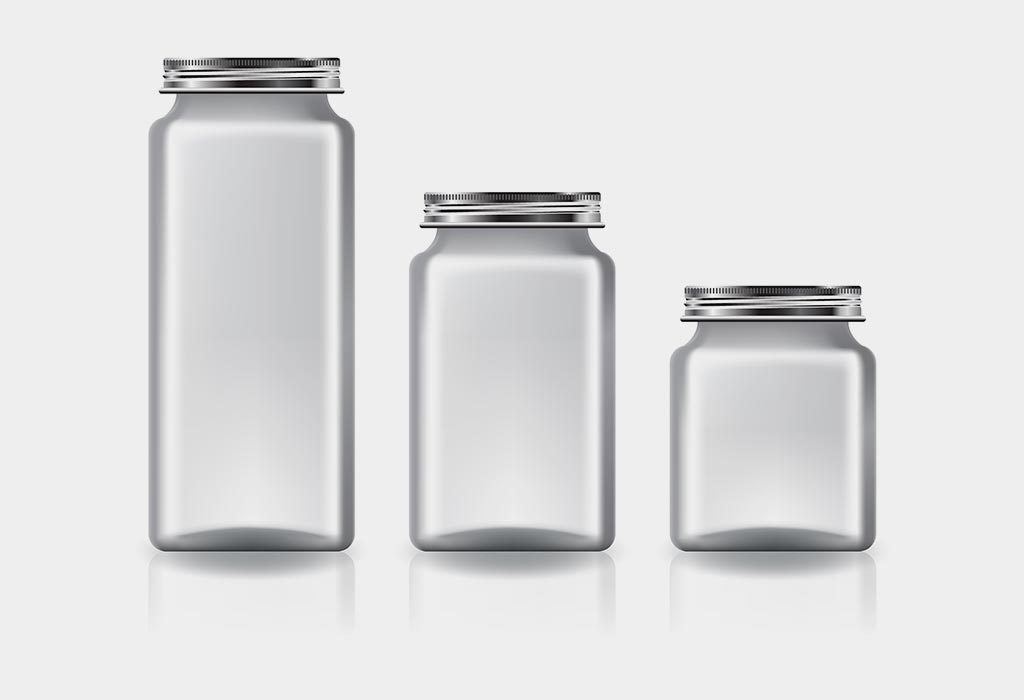### 2. Weight Game

Here is how to teach measurement to grade 1 child in a fun way.

What You Will Need

• Different objects from around the house
• Food weighing scale
• Regular weighing scale

Procedure

• Assemble the items on a table
• Ask your child to choose two items without picking them up
• Separate the two items from the rest and ask your child to guess by looking which is heavier
• Once she has guessed, ask her to hold one in each hand and then ask if her guess is still the same
• Weight the items on the scale to see which one is heavier
• If she guessed wrong, explain to her why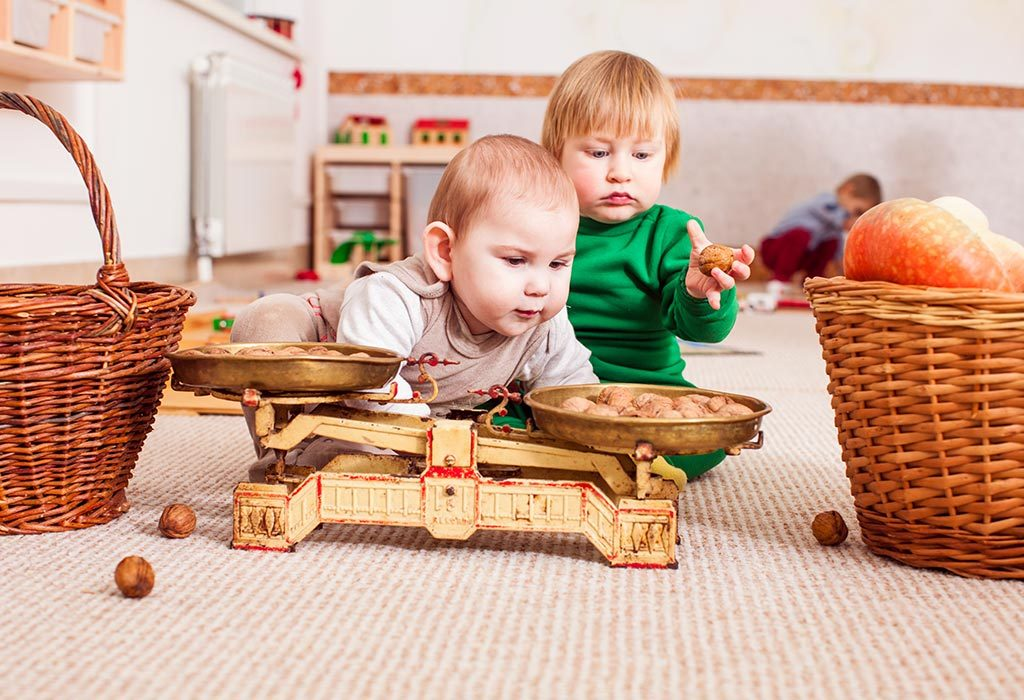### 3. Measure That

Here is one of the fun measuring length activities for preschoolers:

What You Will Need

• Measuring Tapes
• Paper
• Pencil

Procedure

• Make a chart for your children with two columns for “measurement” and “object” and add some objects like “fridge door” “Coffee table” etc
• Show them how to use the measuring tape and have them measure the objects on the list and write the measurements near the objects on the chart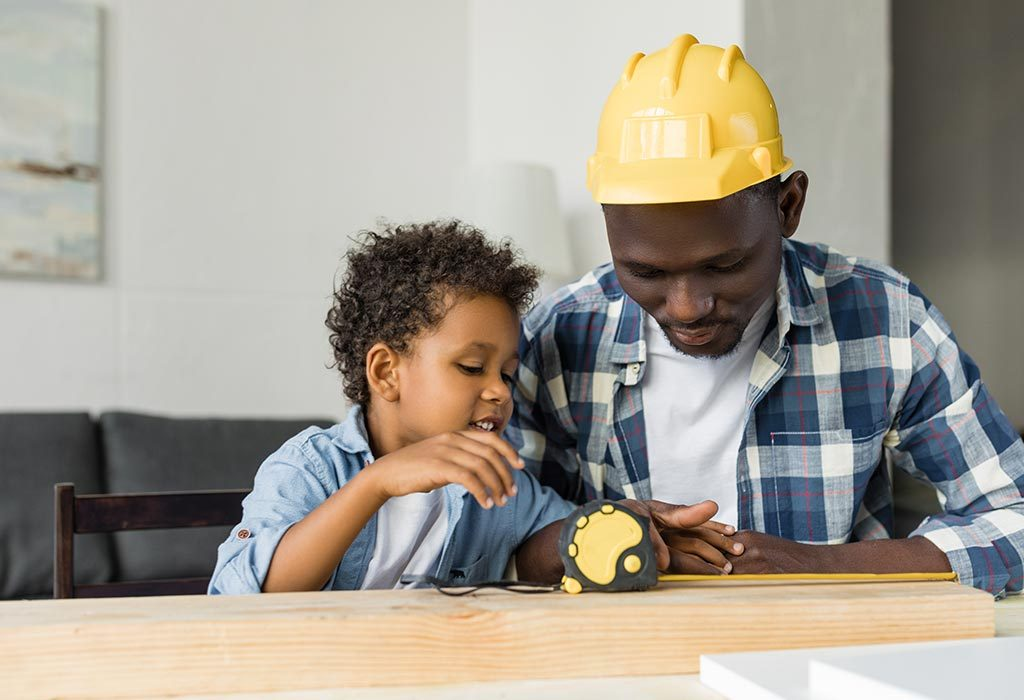### 4. Hot and Cold

Here is one of the fun temperature measurement activities for grade 3:

What You Will Need

• Thermometer
• Five glasses of water; warm, hot, room temperature, cold and iced

Procedure

• Have your child place the thermometer in the bowl of room temperature water and watch the red liquid as it moves. When it stops, they need to write down “Room temperature” and the measurement taken.
• Do the same with the remaining four glasses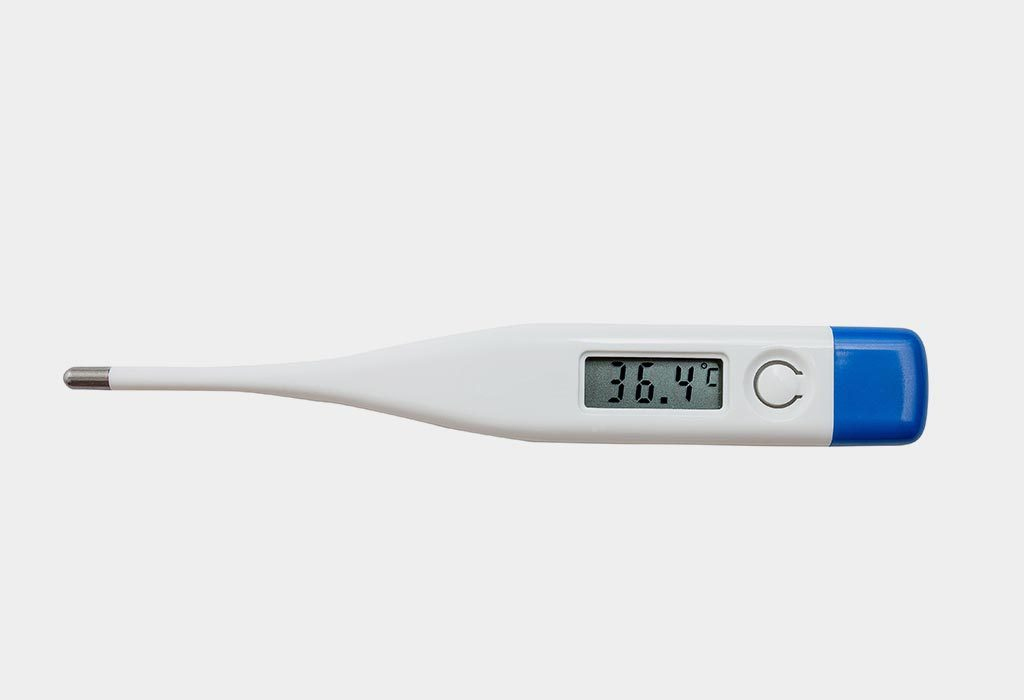### 5. Time For

Learn how to read time with this fun measurement activity for 2nd-grade children:

What You Will Need

• 6 drawn or printed analogue clocks without any hands.
• Pencil

Procedure

• Pick one activity and ask her what time it starts
• Have her draw the hands of the clock to show the starting time
• Have her do the same for the finish time of her activity
• Ask her how many minutes the activity lasted for
• Do the same for the other two activities.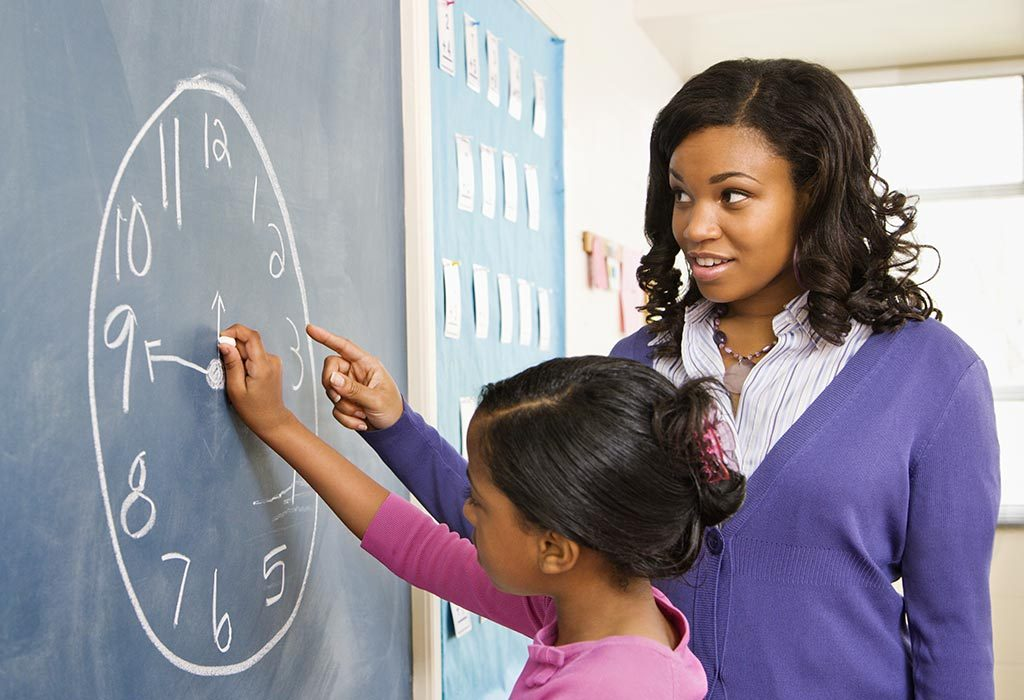Children learn better with hands-on activities, so try out the above activities for a fun experience teaching your children the basics of measurements.

Also Read: Teaching Measurement Concepts to Kids

Previous article «
• RELATED ARTICLES